cancel
Showing results for
Did you mean:## Power BI – Handling Duplicate Rankings Using the RANKX DAX Function

Power BI supports a functional language called DAX (Data Analysis Expressions), which basically represents an executable piece of code inside a function. DAX expressions can sometimes be difficult to use and understand. There are multiple DAX expressions out there, but today I will be concentrating on a problem statement for using the RANKX function, and how to resolve it. The details on this function can be found on Microsoft’s official documentation website: https://docs.microsoft.com/en-us/dax/rankx-function-daxThe task here is to rank customers based on the Purchase date. To achieve this, we can use the RANKX function by writing the ranking calculation as follows: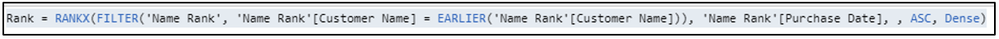The above expression calculates a rank value at a ‘Customer Name’ level using ‘Purchase Date’; in Ascending order and Dense argument allows us to get the next rank value whenever a tie appears. Details on EARLIER function can be found here https://docs.microsoft.com/en-us/dax/earlier-function-dax. We get the following result with a new ‘Rank’ calculation against every customer.Let’s look at the Rank value for a single customer as highlighted above – ‘Alice’ in this case. We see for the instances, that where the purchase date is the same for ‘Alice’, we ended up with same rank, which is logically correct. But what if I want to avoid these duplicate ranks for the same dates for the customer? So, this is the challenge here which we will try to solve.

Let us just display ranks for ‘Alice’: (I have sorted by Purchase date to make the rank values clear):Rank 1 & Rank 2 look perfect, the only issue is the duplicates here. Is there some other column we can add in RANKX to avoid this? In this scenario, I don’t have any other column to further differentiate the ranks. So, how can I resolve this?

Sometimes, an index column in a dataset can be quite useful – in this case we have a column ‘ID’, which serves as an index for the individual rows in the dataset. Let us just create a new rank column as follows: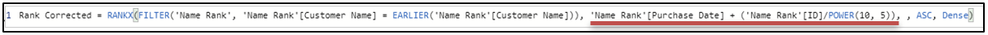Did you notice the difference in this ‘Rank Corrected’ calculation as compared to the ‘Rank’ calculation above? Let’s see what result this gives us: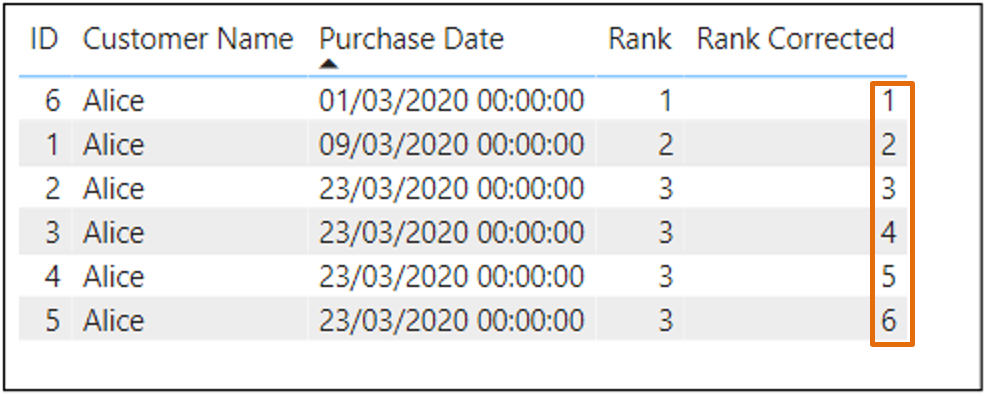We see that the ranks look perfect now without any duplicates. Let me show you what this does. I created a ‘Summation’ column which is just the highlighted part in the ‘Rank Corrected’ calculation.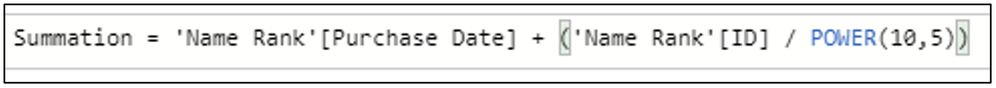Once I move it on my table visual, I see following: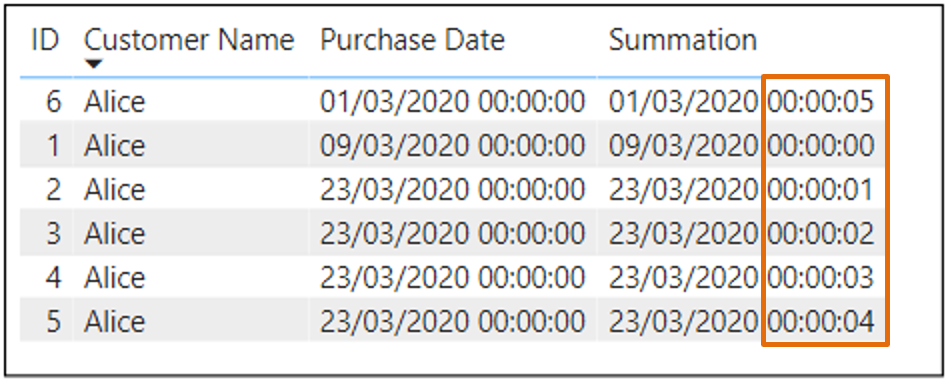Basically, we added a value using an ‘ID’ column to the timestamp part of the ‘Purchase Date’ column and it made a difference in recognising the date values in the scenario where they were the same. The new ‘Rank Corrected’ calculation is calculating rank on this new ‘Summation’ column, which avoids discrepancy around having same values in ‘Purchase Date’ column.

For the 1st row, we see ‘Purchase Date’ = “01/03/2020 00:00:00” and ‘Summation’ = “01/03/2020 00:00:05”. We basically simplified our data for the RANKX expression to perform the ranking calculation more accurately without ending up with duplicate ranks.

Finally, we have got a solution to our problem statement and handled duplicate rankings well. The output for all the customers is shown as follows and they look more promising:Keep Learning & Exploring!

- Pragati